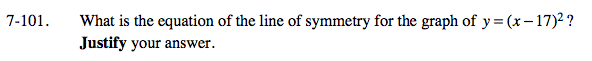### Home > A2C > Chapter 7 > Lesson 7.2.1 > Problem7-101

7-101.

What is the equation of the line of symmetry for the graph of y = (x − 17)2? Justify your answer. Homework Help ✎Where is the vertex?